In :
using BenchmarkTools, Base.Test, PyPlot


# Problem 1: Exponential integrals¶

In this problem, you will write code to compute the exponential integral function $$E_1(z) = \int_z^\infty \frac{e^{-t}}{t} dt$$ For simplicity, you only need to compute it for $\Re z \ge 0$, in which case the definition can be rewritten $$E_1(z) = \int_0^1 \frac{e^{-z/u}}{u} du$$

## Slow version¶

This is the slow, simplistic implementation of this function, from the pset, that directly performs the integral numerically. We will use it for comparisons:

In :
function E₁_slow{T<:AbstractFloat}(z::Union{T,Complex{T}})
real(z) < 0 && error("real(z) < 0 not implemented")
return quadgk(u -> exp(-z/u)/u, 0, 1, reltol=eps(T)*10)
end
E₁_slow{T<:Real}(z::Union{T,Complex{T}}) = E₁_slow(float(z))

Out:
E₁_slow (generic function with 2 methods)

To check this, a useful resource is WolframAlpha, which can compute $E_1(z)$ by ExpIntegralE[1,z]. For $E_1(1.2)$, we get 0.158408436851462561424955970710861738534157976840578963378.... Compared to the above result, this corresponds to a relative error of:

In :
# compute the relative error of approx compared to exact
relerr(approx,exact) = norm(approx - exact) / norm(exact)

relerr(E₁_slow(1.2), 0.158408436851462561424955970710861738534157976840578963378)

Out:
3.5043052210224057e-16

Hooray, we are getting the right result to nearly machine precision! We can even do complex values this way:

In :
relerr(E₁_slow(1.2 + 3.4im),
-0.0196798781439709839467398951111963946354437628483798953 +
0.0708764302707789307217865597073426047954413415009995511im)

Out:
1.886647948886305e-16

## Taylor series¶

The Taylor series for E₁ is: $$E_1(z) = -\log(z) - \gamma - \sum_{k=1}^\infty \frac{(-z)^k}{k!k}$$ where $\gamma$ is the Euler–Mascheroni constant (eulergamma in Julia).

Let's write a function to compute this series until it converges (until the answer stops changing in the current precision), returning the answer and the number of terms required: Important: when computing a series like this, it is important to realize that each term in the series can be computed cheaply from the previous term, rather than computing $z^k/k!$ separately for each term.

In :
function E₁_taylor(z::Number)
E₁ = -eulergamma - log(z)
# iteratively compute the terms in the series, starting with k=1
term = oftype(E₁, z) # use oftype to ensure type stability
E₁ += term
for k=2:1000000
term = -term * z * (k-1) / (k * k)
prev_E₁ = E₁
E₁ += term
E₁ == prev_E₁ && return (E₁, k-1)
end
return E₁, typemax(Int)
end

Out:
E₁_taylor (generic function with 1 method)
In :
relerr(E₁_taylor(1.2), 0.158408436851462561424955970710861738534157976840578963378)

Out:
3.5043052210224057e-16
In :
relerr(E₁_taylor(1.2 + 3.4im),
-0.0196798781439709839467398951111963946354437628483798953 +
0.0708764302707789307217865597073426047954413415009995511im)

Out:
3.3590811736585017e-15

Good, it seems to be giving the correct answer. Now, let's make a plot of how many terms it is requiring to converge:

In :
x = linspace(0,10,200)
pcolor(x',x, [E₁_taylor(x+y*im) for y in x, x in x], cmap="hsv")
colorbar()
xlabel("real(z)")
ylabel("imag(z)")
title(L"number of Taylor-series terms for $E_1(z)$")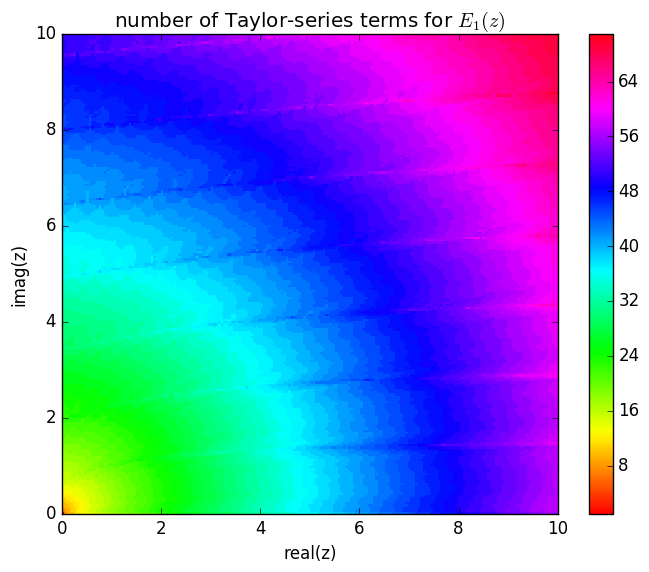Out:
PyObject <matplotlib.text.Text object at 0x3270c6dd0>

Not surprisingly, it is good for small $|z|$ but starts to get big quickly. For large $|z|$, we need a different algorithm, and for that we will use continued fractions.

For very small $|z|$, we will want to just inline the Taylor polynomial with @evalpoly, so let's close by writing a function that just computes the coefficients of the series (not including $\log(z)$):

In :
# SOLUTION code
# n coefficients of the Taylor series of E₁(z) + log(z), in type T:
function E₁_taylor_coefficients{T<:Number}(::Type{T}, n::Integer)
n < 0 && throw(ArgumentError("$n ≥ 0 is required")) n == 0 && return T[] n == 1 && return T[-eulergamma] # iteratively compute the terms in the series, starting with k=1 term::T = 1 terms = T[-eulergamma, term] for k=2:n term = -term * (k-1) / (k * k) push!(terms, term) end return terms end  Out: E₁_taylor_coefficients (generic function with 1 method) In : E₁_taylor_coefficients(Float64, 10)  Out: 11-element Array{Float64,1}: -0.577216 1.0 -0.25 0.0555556 -0.0104167 0.00166667 -0.000231481 2.83447e-5 -3.1002e-6 3.06192e-7 -2.75573e-8  Let's write a macro that inlines this expression for a given number of terms and precision, which we'll use to do performance optimization below. We will only do this Float64 (double) precision, since that is the precision requested by the pset: In : # SOLUTION code macro E₁_taylor64(z, n::Integer) c = E₁_taylor_coefficients(Float64, n) taylor = Expr(:macrocall, Symbol("@evalpoly"), :t, c...) quote let t =$(esc(z))
$taylor - log(t) end end end  Out: @E₁_taylor64 (macro with 1 method) In : relerr(@E₁_taylor64(2.0, 30), E₁_taylor(2.0))  Out: 2.837963777242665e-16 Good, seems to be computing the same thing. ## Continued-fraction expansion¶ The continued-fraction expansion for E₁ is: $$\frac{e^{-z}}{z + \frac{1}{1 + \frac{1}{z + \frac{2}{1 + \frac{2}{z + \frac{3}{1 + \cdots}}}}}}$$ Of course, the exact continued-fraction expansion has an infinite number of terms. If we truncate it to a finite number of terms, there is some ambiguity about what the last term should be (or the first term, since we iterate backwards through the coefficients). People have investigated how to construct and define an optimal truncation for the continued-fraction expansion in some range of parameters, and the result is called a modified approximant [see e.g. equation (≈12) in Backeljaw and Cuyt, "Algorithm 895: A continued fractions package for special functions," ACM TOMS 36, article 15 (2009)]. For E₁, I've played around a bit with this and ended up with a truncation that works pretty well: In : # compute E₁ via n terms of the continued-fraction expansion, implemented # in the simplest way: function E₁_cf(z::Number, n::Integer) # starting with z seems to give many fewer terms for intermediate |z| ~ 3 cf::typeof(inv(z)) = z for i = n:-1:1 cf = z + (1+i)/cf cf = 1 + i/cf end return exp(-z) / (z + inv(cf)) end  Out: E₁_cf (generic function with 1 method) Let's check that this is converging for$z=4$: In : [relerr(E₁_cf(4, n), E₁_slow(4)) for n in 1:20]  Out: 20-element Array{Float64,1}: 0.00582285 0.000476212 5.60469e-5 8.37068e-6 1.48464e-6 3.00369e-7 6.7499e-8 1.65353e-8 4.35523e-9 1.22051e-9 3.60936e-10 1.11899e-10 3.61737e-11 1.21395e-11 4.21339e-12 1.50747e-12 5.54472e-13 2.09189e-13 8.0784e-14 3.17858e-14 The continued-fraction expansion should converge more and more rapidly as$|z|$gets large. Let's try$z = 8$: In : [relerr(E₁_cf(8, n), E₁_slow(8)) for n in 1:20]  Out: 20-element Array{Float64,1}: 0.00162422 6.12415e-5 3.61306e-6 2.87668e-7 2.85331e-8 3.35576e-9 4.52707e-10 6.84218e-11 1.13852e-11 2.05794e-12 3.99931e-13 8.29366e-14 1.83504e-14 4.31774e-15 1.07943e-15 3.59812e-16 1.79906e-16 1.79906e-16 1.79906e-16 1.79906e-16 Now, let's write a function to figure out how many terms we need in this expansion to converge to about 14 digits. (We check convergence by comparing to the cf expansion with twice as many terms.) In : function E₁_cf_nterms(z::Number, reltol=1e-14) for n = 1:100 # give up after 100 terms doubled = E₁_cf(z, 2n) if abs(E₁_cf(z, n) - doubled) ≤ reltol*abs(doubled) return n end end return 101 end  WARNING: Method definition E₁_cf_nterms(Number) in module Main at In:2 overwritten at In:2. WARNING: Method definition E₁_cf_nterms(Number, Any) in module Main at In:2 overwritten at In:2.  Out: E₁_cf_nterms (generic function with 2 methods) In : x = linspace(1,10,100) pcolor(x',x, [E₁_cf_nterms(x+y*im) for y in x, x in x], cmap="hsv") colorbar() xlabel("real(z)") ylabel("imag(z)") title(L"number of cf-series terms for$E_1(z)$")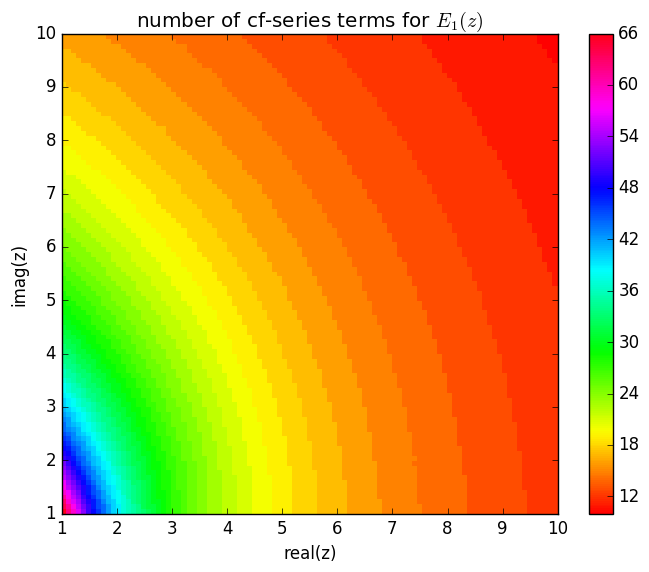Out: PyObject <matplotlib.text.Text object at 0x32e959d10> Notice that the contours can be pretty well approximated by ellipses, similar to the Taylor series above. Now, we'd like to figure out the crossover point where it becomes faster to compute a Taylor series than a continued-fraction expansion. To do this, we need to first come up with a very efficient way to compute the continued-fraction expansion: ### Inlining the continued-fraction expansion for a fixed number of terms:¶ As in pset 2, we may want to inline this whole expansion for a fixed number of terms. It is helpful to be able to look at the expansion, and we'll do this with SymPy as in pset 2: In : using SymPy display("text/latex", E₁_cf(Sym(:z), 4))  $$\frac{e^{- z}}{z + \frac{1}{1 + \frac{1}{z + \frac{2}{1 + \frac{2}{z + \frac{3}{1 + \frac{3}{z + \frac{4}{1 + \frac{4}{z + \frac{5}{z}}}}}}}}}}$$ As in pset 2, we'll use the Polynomials package to write a macro that inlines this as a ratio of two polynomials. (We can't exactly use the code from pset 2, since$z$enters this expansion in a different way than in that pset, but the general idea is the same.) As above, our macro will only work in double (Float64) precision. We can compute the coefficients exactly as integers, but we need to use BigInt because otherwise they will quickly overflow. We can't use BigInt coefficients in the macro, however, because then the resulting computation will be done in (slow!) arbitrary-precision arithmetic, so we convert the coefficients to Float64. In : # SOLUTION code # for numeric-literal coefficients: simplify to a ratio of two polynomials: import Polynomials # return (p,q): the polynomials p(x) / q(x) corresponding to E₁_cf(x, a...), # but without the exp(-x) term function E₁_cfpoly{T<:Real}(n::Integer, ::Type{T}=BigInt) q = Polynomials.Poly(T) p = x = Polynomials.Poly(T[0,1]) for i = n:-1:1 p, q = x*p+(1+i)*q, p # from cf = x + (1+i)/cf = x + (1+i)*q/p p, q = p + i*q, p # from cf = 1 + i/cf = 1 + i*q/p end # do final 1/(x + inv(cf)) = 1/(x + q/p) = p/(x*p + q) return p, x*p + q end macro E₁_cf64(x, n::Integer) p,q = E₁_cfpoly(n, BigInt) evalpoly = Symbol("@evalpoly") num_expr = Expr(:macrocall, evalpoly, :t, Float64.(Polynomials.coeffs(p))...) den_expr = Expr(:macrocall, evalpoly, :t, Float64.(Polynomials.coeffs(q))...) quote let t =$(esc(x))
exp(-t) * $num_expr /$den_expr
end
end
end

WARNING: Method definition E₁_cfpoly(Integer) in module Main at In:6 overwritten at In:6.

Out:
@E₁_cf64 (macro with 1 method)

Let's compare this to SymPy's result for simplifying the continued fraction to a ratio of polynomials, just to make sure we are getting the same polynomial:

In :
display("text/latex", cancel(E₁_cf(Sym(:z), 4)))

$$\frac{z^{5} + 19 z^{4} + 107 z^{3} + 229 z^{2} + 314 z + 250}{z^{6} e^{z} + 20 z^{5} e^{z} + 125 z^{4} e^{z} + 320 z^{3} e^{z} + 480 z^{2} e^{z} + 480 z e^{z} + 120 e^{z}}$$
In :
E₁_cfpoly(4)

Out:
(Poly(250 + 314⋅x + 229⋅x^2 + 107⋅x^3 + 19⋅x^4 + x^5),Poly(120 + 480⋅x + 480⋅x^2 + 320⋅x^3 + 125⋅x^4 + 20⋅x^5 + x^6))

Yay! Finally, these polynomials will be evaluated in the macro by Horner's rule or similar:

In :
display("text/latex", @E₁_cf64 Sym(:z) 4)

$$\frac{\left(z \left(z \left(z \left(z \left(1.0 z + 19.0\right) + 107.0\right) + 229.0\right) + 314.0\right) + 250.0\right) e^{- z}}{z \left(z \left(z \left(z \left(z \left(1.0 z + 20.0\right) + 125.0\right) + 320.0\right) + 480.0\right) + 480.0\right) + 120.0}$$

Although the transformation of the continued fraction to a ratio of polynomials is exact in the absence of roundoff errors, we should double check that this hasn't accidentally created big rounding errors:

In :
semilogy(0:30, [relerr(@eval(@E₁_cf64(3.0, $n)), E₁_cf(3.0, n)) for n in 0:30], "ro") xlabel("order n") ylabel("relative error")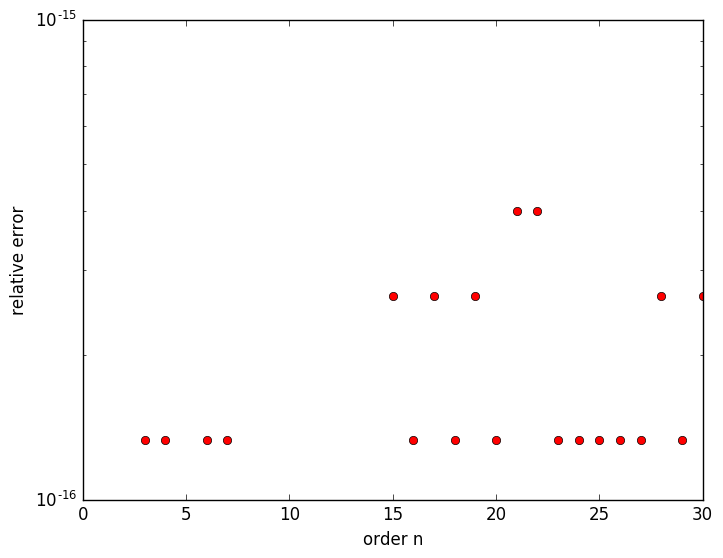Out: PyObject <matplotlib.text.Text object at 0x333cbbad0> Looks good! ## Taylor/continued-fraction crossover¶ Let's do some benchmarking to figure out (roughly) at what$|z|$it becomes cheaper to use a hard-coded continued-fraction expansion than a hard-coded Taylor expansion: In : function time_taylor(z) E₁, n = E₁_taylor(z) f = gensym() # generate a function name so that we can benchmark in a function @eval$f(z) = @E₁_taylor64 z $n b = @eval @benchmark$f($z) return time(minimum(b)) # time in ns end function time_cf(z) n = E₁_cf_nterms(z) f = gensym() # generate a function name so that we can benchmark in a function @eval$f(z) = @E₁_cf64 z $n b = @eval @benchmark$f($z) return time(minimum(b)) # time in ns end  WARNING: Method definition time_taylor(Any) in module Main at In:2 overwritten at In:2. WARNING: Method definition time_cf(Any) in module Main at In:9 overwritten at In:9.  Out: time_cf (generic function with 1 method) In : x = linspace(2,5, 10) semilogy(x, [time_taylor(x) for x in x], "ro-") semilogy(x, [time_cf(x) for x in x], "bs-") xlabel("real(z)") ylabel("time (ns)") legend(["taylor", "cf"]) title("timing comparison for inlined Taylor vs. cf (real z)")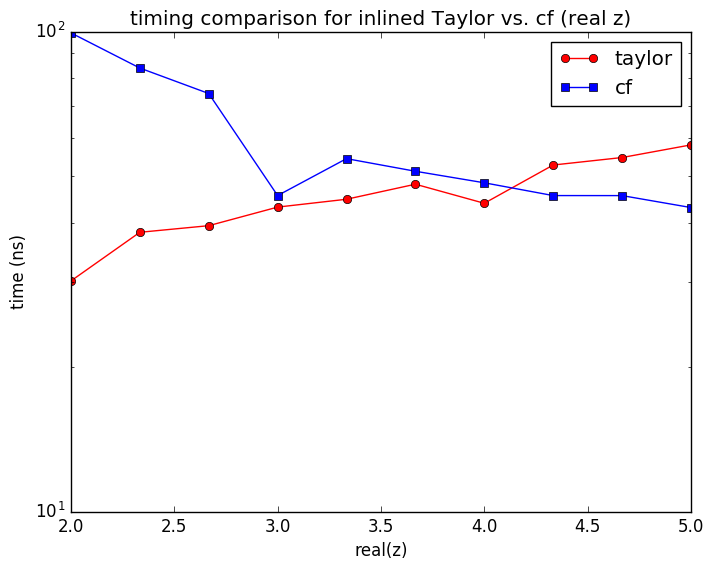Out: PyObject <matplotlib.text.Text object at 0x32f0ada10> In : x = linspace(2,5, 10) semilogy(x, [time_taylor(x + 0im) for x in x], "ro-") semilogy(x, [time_cf(x + 0im) for x in x], "bs-") xlabel("real(z)") ylabel("time (ns)") legend(["taylor", "cf"]) title("timing comparison for inlined Taylor vs. cf (complex z)")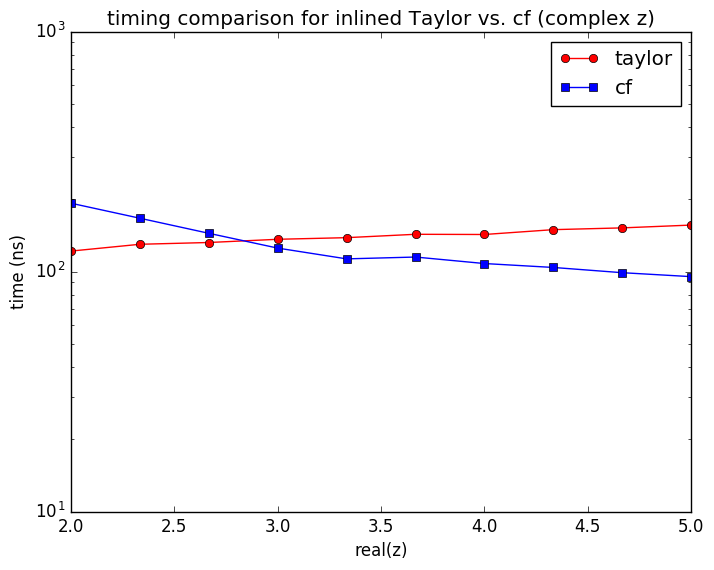Out: PyObject <matplotlib.text.Text object at 0x32f379490> In : x = linspace(3,8, 10) semilogy(x, [time_taylor(x*im) for x in x], "ro-") semilogy(x, [time_cf(x*im) for x in x], "bs-") xlabel("real(z)") ylabel("time (ns)") legend(["taylor", "cf"]) title("timing comparison for inlined Taylor vs. cf (imaginary z)")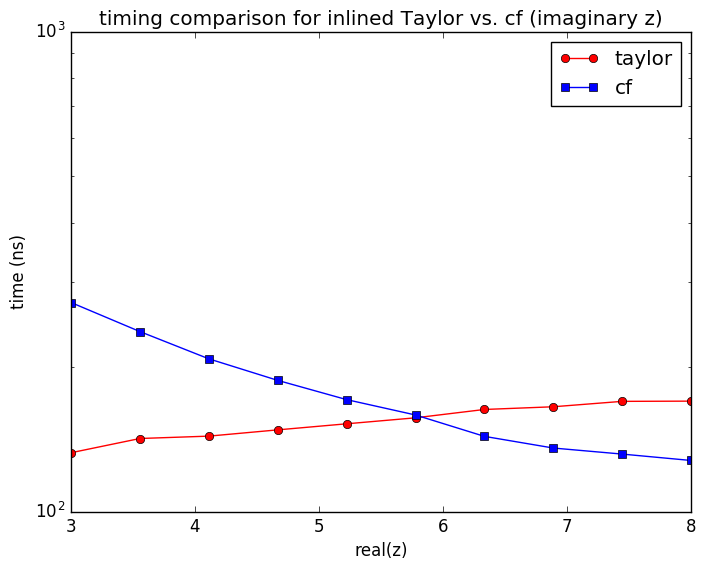Out: PyObject <matplotlib.text.Text object at 0x32f99f350> So, it looks like the inlined contined-fraction approach becomes better than Taylor at around$z \approx 3$on the real-$z$and about$z \approx 5$on the imaginary-$z$axis, where the number of terms in both the cf and Taylor expansions is about 30: In : E₁_cf_nterms(3.0), E₁_cf_nterms(5.0im)  Out: (26,33) In : E₁_taylor(3.0), E₁_taylor(5.0im)  Out: (28,35) ## Putting it all together¶ Let's put this all together into a single function that splits the (right-half) complex plane into different ellipses and uses hard-coded Taylor or continued-fraction expansions in each piece. First, let's figure out the dividing lines along the real and imaginary axes to use continued-fraction expansions with 30, 15, 8, and 4 terms: In : x = 1:0.2:1000 cf_cutoffs = hcat([ x[findfirst(x -> E₁_cf_nterms(x) < n, x)] for n in (30,15,8,4) ], [ x[findfirst(x -> E₁_cf_nterms(x*im) < n, x)] for n in (30,15,8,4) ])  Out: 4×2 Array{Float64,2}: 2.8 5.8 7.6 12.0 23.2 28.8 199.0 203.6 If we approximate the cutoff in the complex plane$z=x + iy$by an ellipse, then we want an equation$x^2 + ay^2 ≥ b^2$, where$a$and$b$are given by: In : hcat( (cf_cutoffs[:,1]./cf_cutoffs[:,2]).^2, cf_cutoffs[:,1].^2 )  Out: 4×2 Array{Float64,2}: 0.233056 7.84 0.401111 57.76 0.64892 538.24 0.955324 39601.0  Similarly for the Taylor expansion, except that here we need to look for very small$x$, and we want the largest$x$rather than the smallest: In : x = logspace(-8,1,10000) taylor_cutoffs = hcat([ x[findlast(x -> E₁_taylor(x) < n, x)] for n in (30,15,8,4) ], [ x[findlast(x -> E₁_taylor(x*im) < n, x)] for n in (30,15,8,4) ])  Out: 4×2 Array{Float64,2}: 3.36863 3.52578 0.605589 0.633841 0.0539229 0.0539229 0.00045401 0.00045401 In : hcat( (taylor_cutoffs[:,1]./taylor_cutoffs[:,2]).^2, taylor_cutoffs[:,1].^2 )  Out: 4×2 Array{Float64,2}: 0.912843 11.3476 0.912843 0.366738 1.0 0.00290768 1.0 2.06125e-7 However, this is not quite good enough along the imaginary axis: 30 terms in the Taylor series is fine up to$\Im z ≤ 3.53$, but 30 terms in the cf expansion was good for$\Im z ≥ 5.8$. We need to figure out how many terms in the Taylor series we need to go up to$\Im z ≤ 5.8$: In : E₁_taylor(5.8*im)  Out: 37 Also, for large enough$\Re z$, the E₁ function will underflow: give 0.0 because the result is smaller than the minimum representable floating-point number. This will happen quite quickly for large$\Re z$because of the$e^{-z}$dependence. Let's find out when this occurs on the real axis: In : x = linspace(1,2000,10000) x[findfirst(x -> E₁_cf(x,0) == 0.0, x)]  Out: 738.7047704770478 In : E₁_cf(739 - 4im,0)  Out: -0.0 - 0.0im In : 739^2  Out: 546121 Finally, we'll hard-code our double-precision E₁ function using these dividing points, assuming that they describe the semi-axes of ellipses in the complex$z$plane (as seems to be the case from our numerical experiments above): In : # SOLUTION: function E₁(z::Union{Float64,Complex{Float64}}) x² = real(z)^2 y² = imag(z)^2 if x² + 0.233*y² ≥ 7.84 # use cf expansion, ≤ 30 terms if (x² ≥ 546121) & (real(z) > 0) # underflow return zero(z) elseif x² + 0.401*y² ≥ 58.0 # ≤ 15 terms if x² + 0.649*y² ≥ 540.0 # ≤ 8 terms x² + y² ≥ 4e4 && return @E₁_cf z 4 return @E₁_cf64 z 8 end return @E₁_cf64 z 15 end return @E₁_cf64 z 30 else # use Taylor expansion, ≤ 37 terms r² = x² + y² return r² ≤ 0.36 ? (r² ≤ 2.8e-3 ? (r² ≤ 2e-7 ? @E₁_taylor64(z,4) : @E₁_taylor64(z,8)) : @E₁_taylor64(z,15)) : @E₁_taylor64(z,37) end end E₁{T<:Integer}(z::Union{T,Complex{T},Rational{T},Complex{Rational{T}}}) = E₁(float(z))  WARNING: Method definition E₁(Union{Float64, Base.Complex{Float64}}) in module Main at In:3 overwritten at In:3.  Out: E₁ (generic function with 2 methods) For accuracy-testing purposes, let's compare it to a function that uses 50 terms in Taylor or cf: In : E₁_test(z::Union{Float64,Complex{Float64}}) = real(z)^2 + imag(z)^2 > 6 ? E₁_cf(z,100) : E₁_taylor(z)  WARNING: Method definition E₁_test(Union{Float64, Base.Complex{Float64}}) in module Main at In:1 overwritten at In:1.  Out: E₁_test (generic function with 1 method) In : x = linspace(1e-10,10,100) pcolor(x',x, [log10(1e-16 + relerr(E₁(x+y*im),E₁_test(x+y*im))) for y in x, x in x], cmap="cool") colorbar() xlabel("real(z)") ylabel("imag(z)") title(L"$\log_{10}$relative error in optimized$E_1(z)$")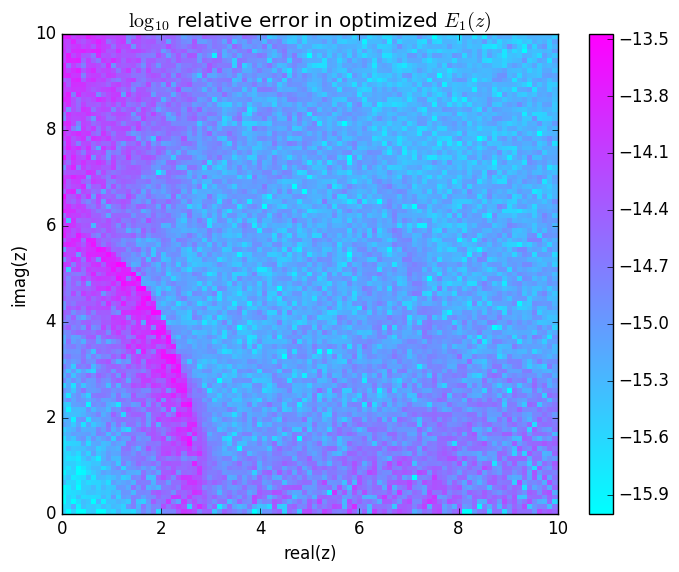Out: PyObject <matplotlib.text.Text object at 0x338b1aa10> It seems to be achieving the required 13 digits of accuracy specified in the pset. However, if we were really serious about this, we should probably look at alternative expansions to get better accuracy in the purple regions of the plot above. (The simplest thing might be to do a Taylor expansion around, say,$z=2+4i$. There are also alternative forms of the continued-fraction expansion and other identities that we could try to exploit.) Now let's compare performance at a few points versus our original quadgk implementation: In : @btime E₁(2+2im) @btime E₁_slow(2+2im)   193.599 ns (1 allocation: 32 bytes) 44.889 μs (425 allocations: 10.55 KiB)  Out: -0.033767089606562 - 0.01859941416975054im In : @btime E₁(10+2im) @btime E₁_slow(10+2im)   139.873 ns (1 allocation: 32 bytes) 18.827 μs (183 allocations: 4.61 KiB)  Out: -2.3461694530923403e-6 - 3.347026042268865e-6im In : @btime E₁(5) @btime E₁_slow(5)   109.531 ns (1 allocation: 16 bytes) 8.282 μs (159 allocations: 3.45 KiB)  Out: 0.001148295591275326 This is almost a factor of 100 speedup even in cases where the expansion requires a lot of terms, which seems pretty good. It would be good to also compare to a highly optimized implementation, e.g. the exp1 function in SciPy. Because that is a Python function, we need to benchmark it for a whole array of numbers in order to make a reasonable comparison: In : using PyCall exp1 = pyimport_conda("scipy.special", "scipy")["exp1"]  Out: PyObject <ufunc 'exp1'> In : relerr(E₁(2+2im), exp1(2+2im))  Out: 5.75981106819841e-15 In : z = rand(10^6)*10 + rand(10^6)*10im @btime pycall($exp1, PyObject, $(PyObject(z))) evals=1;   1.282 s (12 allocations: 400 bytes)  In : E₁(z::AbstractVector) = map(E₁, z) @btime E₁($z) evals=1;

  209.063 ms (1000005 allocations: 45.78 MiB)


In :
@btime pycall($exp1, PyObject,$(PyObject(real(z)))) evals=1;
@btime E₁($(real(z))) evals=1;   503.554 ms (12 allocations: 400 bytes) 102.485 ms (1000005 allocations: 22.89 MiB)  Pretty good! We are 6 times faster than the SciPy routine for complex arguments, and 5 times faster for real arguments, even though SciPy internally calls an optimized Fortran routine. It's actually not unusual for special functions written in Julia to beat older optimized C and Fortran implementations. We've observed this for the erfinv and polygamma functions, for example. The reason is that the inlining performed by macros like @evalpoly and @E₁_cf64 is hard to replicate in lower-level languages — hardly anyone bothers to write the code-generation programs that would be required — but it is fairly easy in Julia. # Problem 2: Parallel mapreduce¶ Our task is to speed up the function myreduce(⊕, n) that computes $$1 \oplus 2 \oplus 3 \oplus \cdots \oplus n$$ for$n \ge 0$, where$\oplus\$ is a caller-supplied commutative binary function ⊕(x,y), and we are allowed to assume commutativity.

A simple parallel solution for this is similar to the pmap function described in the Julia manual: we just ask the worker processes to "add" (⊕) terms one by one, giving it another term to add as soon as the worker becomes idle.

In :
function preduce(⊕, lst::AbstractVector)
if nprocs() == 1
return reduce(⊕, lst)
end
n = length(lst)
queue = Base.copymutable(lst)
@sync begin
for p in workers()
@async begin
while length(queue) > 1
a = pop!(queue)
b = pop!(queue)
# compute results[p] = results[p] ⊕ lst[idx]
push!(queue, remotecall_fetch(⊕, p, a, b))
end
end
end
end
if isempty(queue)
return ⊕() # return empty call if it is defined
else
assert(length(queue) == 1)
return queue
end
end
preduce(⊕, n::Integer) = preduce(⊕, 1:n)

WARNING: Method definition preduce(Any, AbstractArray{T<:Any, 1}) in module Main at In:2 overwritten at In:2.
WARNING: Method definition preduce(Any, Integer) in module Main at In:26 overwritten at In:26.

Out:
preduce (generic function with 2 methods)
In :
preduce(+, 1000) == 500500

Out:
true
In :
function weirdplus(x,y)
sleep(1e-3 / (x+y)) # wait for some number of seconds
return x + y
end
preduce(weirdplus, 1000)

WARNING: Method definition weirdplus(Any, Any) in module Main at In:2 overwritten at In:2.

Out:
500500

Now, let's check how well this paralellizes:

In :
@btime preduce(weirdplus, 1000) evals=1

  1.376 s (6065 allocations: 377.69 KiB)

Out:
500500
In :
addprocs(4) # parallelize over 4 workers
workers()

Out:
4-element Array{Int64,1}:
14
15
16
17
In :
@everywhere function weirdplus(x,y)
sleep(1e-3 / (x+y)) # wait for some number of seconds
return x + y
end
@btime preduce(weirdplus, 1000) evals=1

WARNING: Method definition weirdplus(Any, Any) in module Main at In:2 overwritten at In:2.

  434.293 ms (92426 allocations: 3.76 MiB)

Out:
500500
In :
1.376 / 0.434293

Out:
3.1683678990911663

Not terrible, a 3x speedup.

One limitation of the preduce solution above is that it assumes that evaluations of ⊕ are individually quite expensive, so that the overhead of one remotecall_fetch per call to ⊕ is not important.

If ⊕ were a very cheap function, like +, this would be terrible! There are various approaches that we could pursue to improve this, like iteratively doubling the number of elements that are sent to the workers until the time per remotecall_fetch exceeds some threshold, but I won't attempt to implement them here.